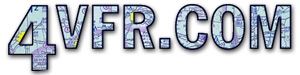Articles | Index

Standard Rate Turns
2010-03-18All banks under the hood are at standard rate. Standard rate turns requires two minutes to make a 360 degree circle. One minute for a 180 and 30 seconds for each 90 degrees. The standard rate turn for a given airspeed is indicated by the needle or by the turn coordinator. The turn coordinator is the only true indicator of whether the bank is left or right. (A necessary check during spins) The angle can be estimated by dividing the speed by ten and adding five. (Speed /10 + 5 = angle of bank) 85 knots airspeed divided by 10 is 8.5 + 5 = 13.5 angle of bank. Set the estimated angle on the AI and check with the turn coordinator. All climbs and descents are at one bar width on the attitude indicator. From low cruise both climb and descent at a given rate will closely correspond to RPM changes by 100's. A power reduction of 500 rpm gives 500 fpm descent.

Comment